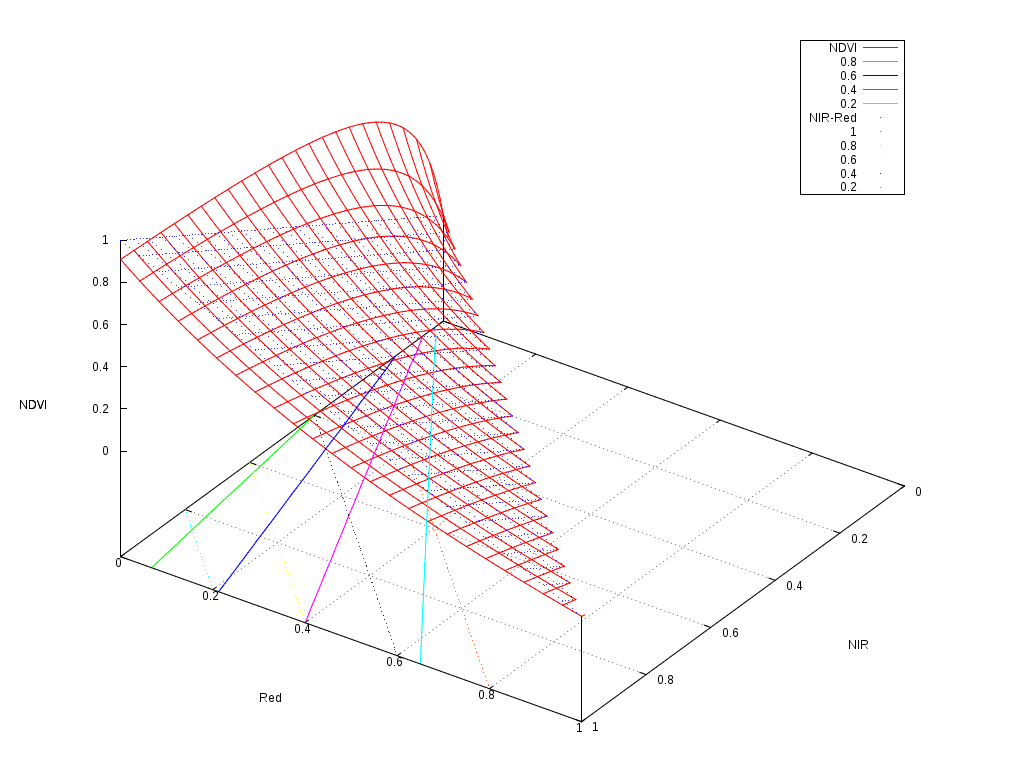17 Dec 2014

# On the usefulness of non-linear indices for remote sensing image classification

Not long ago, after a presentation about satellite image time series classification for land cover map production, someone asked me about the real interest of using non-linear radiometric indices as features for the classification.

By non-linear radiometric indices, I mean non-linear combinations of the reflectances of the different spectral bands. The most current example is the NDVI:

$NDVI = \frac{NIR-Red}{NIR+Red}$

Many other examples are listed in the Orfeo Toolbox wiki and they are useful for vegetation, but also for other kinds of surfaces like water, bare soils, etc.

The question is really interesting, since if we use a “good enough” classifier, it should be able to efficiently use the spectral reflectances without needing us to find clever combinations of them.

Actually, in terms of information theory, the amount of information given to the classifier is exactly the same if we provide it with the reflectances or with the reflectances and non-linear combinations of them.

The fact is that many classifiers, like decision trees or linear SVM, are only able to perform linear or piece-wise linear separations in the feature space, and therefore, non-linear combinations of the input features should help them.

On the other hand, most of these non-linear indices follow the same template as the NDVI: they are normalised differences of 2 bands. Therefore, the non-linearity is not very strong.

The following figure shows the shape of the NDVI as a function of the 2 reflectances used to compute it.As one can see, it follows a plane with a slight non-linearity for the low reflectances. The contour plots are the solid colour lines, which are rather linear. So why we find that classifications often work better when we use this kind of indices?

The answer seems to reside in the distortion of the dynamics of the values.

If we have a look at the floor of the picture, we can imagine how a classifier would work.

The simplest classifier, that is a binary thresholding of the reflectances could only build a decision function following the black dotted grid, that is boundaries perpendicular to the axis. A decision tree, is just a set of thresholds on the features, and therefore, it could model a decision function which would be composed of a step functions on this plane, but always perpendicular and parallel to the axis.

On the other hand, a linear perceptron or a linear SVM, could build a decision function which would correspond to any straight line crossing this plane. An example of these lines would be the colour dotted lines representing the $$NIR-Red$$ values. Any linear combination of the features would work like this.

It seems therefore, that a linear classifier could draw a boundary between a pair of solid colour lines, and therefore efficiently split the feature space as if the NDVI was available.

The interesting thing to note is that the NDVI contour plots are nearly straight lines, but they are not parallel. This introduces a kind of non-linear normalisation of the features which makes the job of the classifier easier.

And what about non-linear classifiers like multi-layer perceptrons or Gaussian SVM? Do they need these non-linear indices? The theoretical answer is “no, they don't”. But the pragmatic answer should be “please, be nice to your classifier”.

Most of the learning algorithms in the literature are based on the optimisation of cost functions. The easier the problem to solve, the higher the chances to get a robust classifier. In the case of SVM, this will result into a larger margin. In the case of Neural Networks, this will lead to a lesser chance of over-fitting.

Another thing to take into account is that cost functions in machine learning problems are based on distances, and most of the time the Euclidean distance is used. It is therefore useful to tune the features in order to give them the appropriate weight into this distance. In other words, if vegetation presence is something which conveys useful information for the classification, use the NDVI so that your classifier knows that1.

Finally, non-linear classifiers are usually more computationally expensive than linear ones, both at the learning and at the inference step. Therefore, it is usually more effective to put the intelligence in the design of the features and use a simpler classifier than using raw features and a very sophisticated classifier.

Of course, all this advice is derived from a mix of the knowledge of the algorithms and the experience in using them for a particular kind of problem: remote sensing image supervised classification for land cover map production. For other applications in computer vision (like object recognition) or other completely unrelated fields, the advice might be different.

## Footnotes:

1

Some call this prior knowledge, others call it common sense.